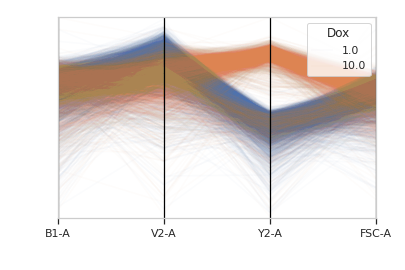# cytoflow.views.parallel_coords¶

class cytoflow.views.parallel_coords.ParallelCoordinatesView[source]

Plots a parallel coordinates plot. PC plots are good for multivariate data; each vertical line represents one attribute, and one set of connected line segments represents one data point.

channels

The channels to view

Type: List(Str)
scale

Re-scale the data in the specified channels before plotting. If a channel isn’t specified, assume that the scale is linear.

Type: Dict(Str : {“linear”, “logicle”, “log”})
xfacet, yfacet

Set to one of the conditions in the Experiment, and a new row or column of subplots will be added for every unique value of that condition.

Type: String
huefacet

Set to one of the conditions in the in the Experiment, and a new color will be added to the plot for every unique value of that condition.

Type: String
huescale

How should the color scale for huefacet be scaled?

Type: {‘linear’, ‘log’, ‘logicle’}

Examples

Make a little data set.

>>> import cytoflow as flow
>>> import_op = flow.ImportOp()
>>> import_op.tubes = [flow.Tube(file = "Plate01/RFP_Well_A3.fcs",
...                              conditions = {'Dox' : 10.0}),
...                    flow.Tube(file = "Plate01/CFP_Well_A4.fcs",
...                              conditions = {'Dox' : 1.0})]
>>> import_op.conditions = {'Dox' : 'float'}
>>> ex = import_op.apply()


Plot the PC plot.

>>> flow.ParallelCoordinatesView(channels = ['B1-A', 'V2-A', 'Y2-A', 'FSC-A'],
...                              scale = {'Y2-A' : 'log',
...                                       'V2-A' : 'log',
...                                       'B1-A' : 'log',
...                                       'FSC-A' : 'log'},
...                              huefacet = 'Dox').plot(ex)plot(experiment, **kwargs)[source]

Plot a faceted parallel coordinates plot

Parameters: experiment (Experiment) – The Experiment to plot using this view. title (str) – Set the plot title xlabel, ylabel (str) – Set the X and Y axis labels huelabel (str) – Set the label for the hue facet (in the legend) legend (bool) – Plot a legend for the color or hue facet? Defaults to True. sharex, sharey (bool) – If there are multiple subplots, should they share axes? Defaults to True. row_order, col_order, hue_order (list) – Override the row/column/hue facet value order with the given list. If a value is not given in the ordering, it is not plotted. Defaults to a “natural ordering” of all the values. height (float) – The height of each row in inches. Default = 3.0 aspect (float) – The aspect ratio of each subplot. Default = 1.5 col_wrap (int) – If xfacet is set and yfacet is not set, you can “wrap” the subplots around so that they form a multi-row grid by setting col_wrap to the number of columns you want. sns_style ({“darkgrid”, “whitegrid”, “dark”, “white”, “ticks”}) – Which seaborn style to apply to the plot? Default is whitegrid. sns_context ({“paper”, “notebook”, “talk”, “poster”}) – Which seaborn context to use? Controls the scaling of plot elements such as tick labels and the legend. Default is talk. despine (Bool) – Remove the top and right axes from the plot? Default is True. min_quantile (float (>0.0 and <1.0, default = 0.001)) – Clip data that is less than this quantile. max_quantile (float (>0.0 and <1.0, default = 1.00)) – Clip data that is greater than this quantile. lim (Dict(Str : (float, float))) – Set the range of each channel’s axis. If unspecified, assume that the limits are the minimum and maximum of the clipped data alpha (float (default = 0.02)) – The alpha blending value, between 0 (transparent) and 1 (opaque). axvlines_kwds (dict) – A dictionary of parameters to pass to ax.axvline

Notes

This uses a low-level API for speed; there are far fewer visual options that with other views.